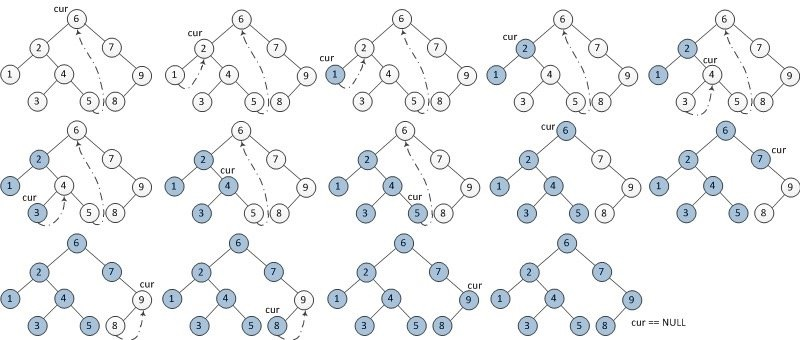12345678 输入: [1,null,2,3] 1 \ 2 / 3 输出: [1,3,2] 

 123456 # Definition for a binary tree node. # class TreeNode: # def __init__(self, x): # self.val = x # self.left = None # self.right = None 

### 思路一

 1234567891011 class Solution: def inorderTraversal(self, root: TreeNode) -> List[int]: def backtrack(p): if p is None: return backtrack(p.left) rs.append(p.val) backtrack(p.right) rs = [] backtrack(root) return rs 

### 思路二

 1234567891011 class Solution: def inorderTraversal(self, root: TreeNode) -> List[int]: rs, stack, cur = [], [], root while cur or stack: while cur: stack.append(cur) cur = cur.left cur = stack.pop() rs.append(cur.val) cur = cur.right return rs 

### 思路三

Morris Traversal 遍历。时间复杂度 $O(n)$，额外空间复杂度 $O(1)$。该方法需要找到当前结点的前驱结点。步骤如下

1. 如果当前节点的左孩子为空，则输出当前节点并将其右孩子作为当前节点。
2. 如果当前节点的左孩子不为空，在当前节点的左子树中找到当前节点在中序遍历下的前驱节点。
1. 如果前驱节点的右孩子为空，将它的右孩子设置为当前节点。当前节点更新为当前节点的左孩子。
2. 如果前驱节点的右孩子为当前节点，将它的右孩子重新设为空（恢复树的形状）。输出当前节点。当前节点更新为当前节点的右孩子。
3. 重复以上1、2直到当前节点为空。1234567891011121314151617181920 class Solution: def inorderTraversal(self, root: TreeNode) -> List[int]: rs, cur, pre = [], root, None while cur: if cur.left is None: rs.append(cur.val) cur = cur.right else: # 寻找当前结点的前驱结点 pre = cur.left while pre.right and pre.right != cur: pre = pre.right if pre.right is None: pre.right = cur cur = cur.left else: pre.right = None # 恢复树的形状 rs.append(cur.val) cur = cur.right return rs